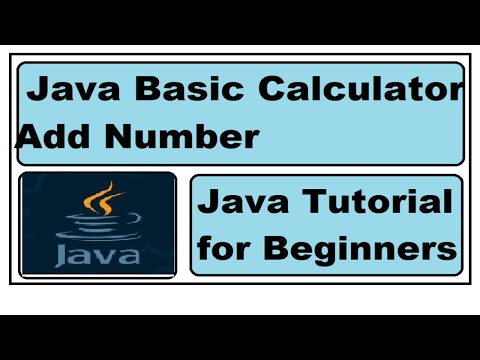## Wednesday, December 14, 2022

### Assignment of Arithmetic Operators Java | Tutorial for Beginners

In this tutorial you will learn how to create and call non static method . We will create method and do operation of addition, subtraction, multiplication,  and division. If you want video tutorial here is link in English and in Hindi language. So lets create some basic math calculator.

Java Tutorial in English

Java Tutorial in Hindi

Lets create class and and check mark on public static void main so it will create main method where we call .

Now we will create method .we used void since we do not want to return but just print . After method we will use bracket and then curly brace and we will write our logic here. we will create variable a and b and provide value, since we want to add we created another variable name result and we will adding a and b since this hold value of first and second value. And finally we want to print this but we only created non static method but not calling . But we now in main method since it is entry point.

int a = 2;

int b =3;

int result =(a+b);

System.out.println(result);

}

Syntax of calling non static method first we will type name of class and then name variable then equal sign for assignment and new word, then class name and bracket. Since we have created object. Now type that and use dot now will get list of all method which we can access.  We select our method then click on run and check output.

Now we will create method and we will change assignment for subtraction, (a -b) and we observe in output, Now we will do for multiplication and division .

If you want to watch  more video click on